# Visualizing power spectral density using Obspy in Python (codes included)

Short demonstration of the ppsd class defined in Obspy using 3 days of data for station PB-B075

For details, visit Visualizing Probabilistic Power Spectral Densities.

## Contents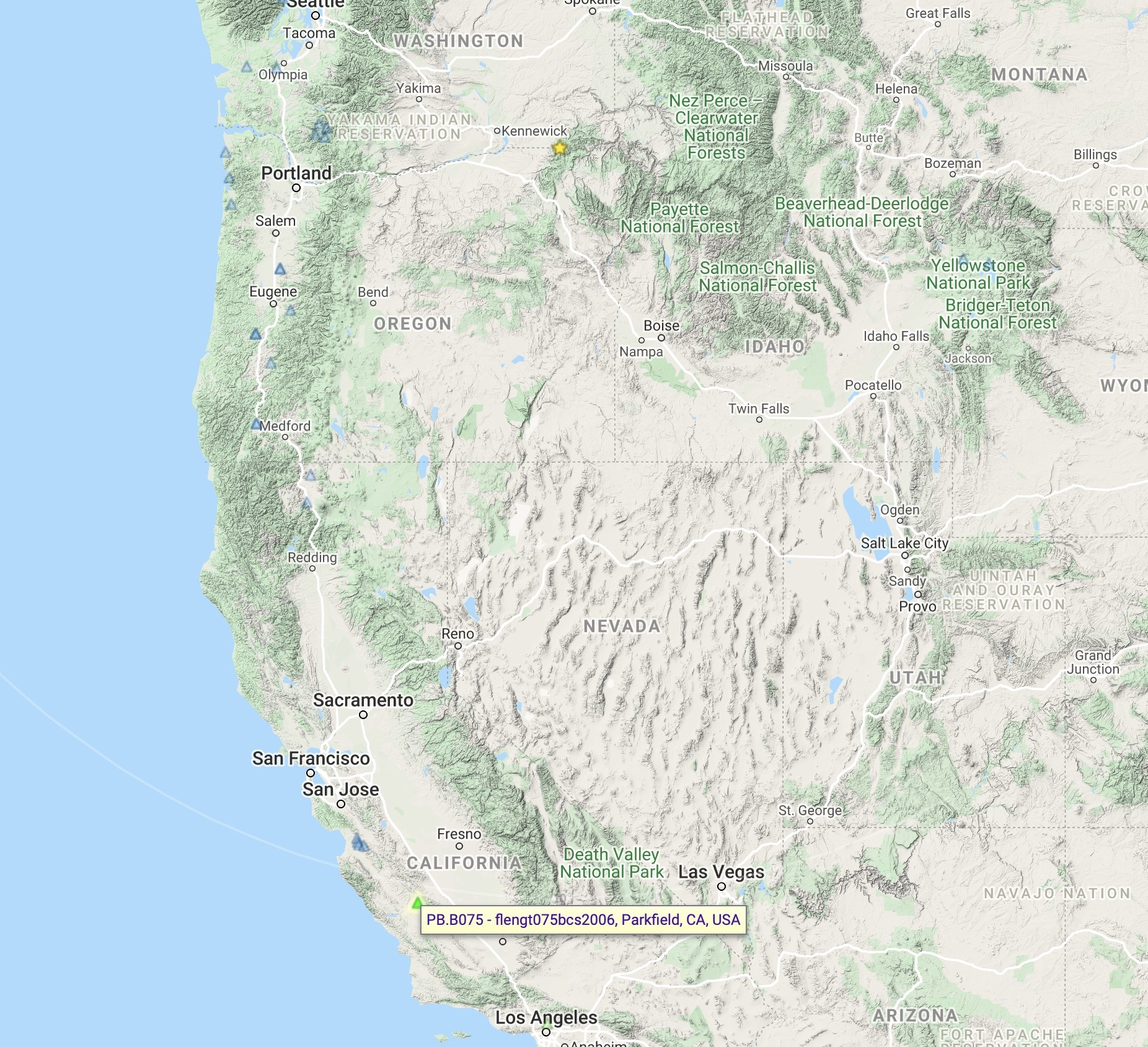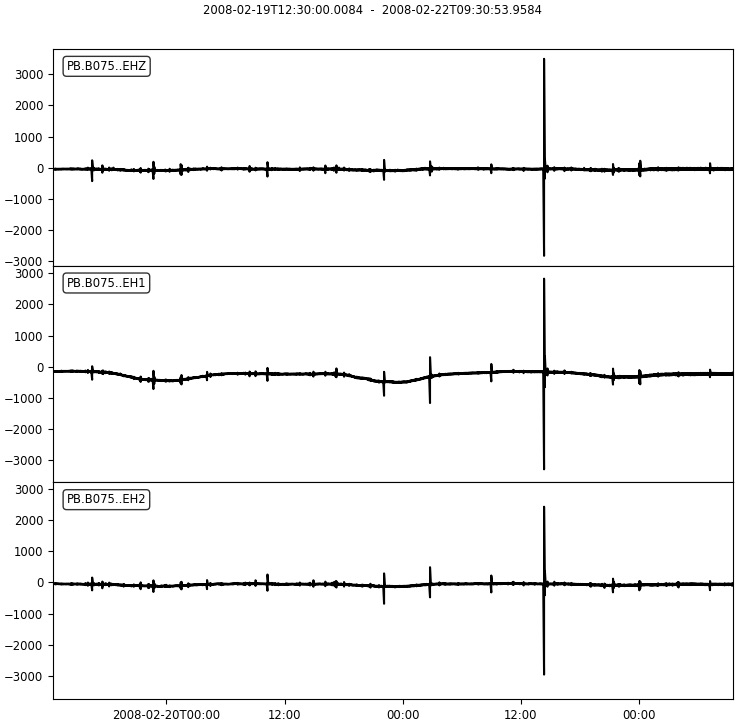## Similar posts

### Import necessary libraries

``````from obspy.io.xseed import Parser
from obspy.signal import PPSD
from obspy.clients.fdsn import Client
import os, glob
import matplotlib.pyplot as plt
from obspy.imaging.cm import pqlx
import warnings
warnings.filterwarnings('ignore')
``````

``````## Downloading inventory
net = 'PB'
sta = 'B075'
loc='*'
chan = 'EH*'
filename_prefix = f"{net}_{sta}"
mseedfiles = glob.glob(filename_prefix+".mseed")
xmlfiles = glob.glob(filename_prefix+'_stations.xml')

if not len(mseedfiles) or not len(xmlfiles):
time = UTCDateTime('2008-02-19T13:30:00')
wf_starttime = time - 60*60
wf_endtime = time + 3 * 24 * 60 * 60 #3 days of data (requires atleast 1 hour)

client = Client('IRIS')

st = client.get_waveforms(net, sta, loc, chan, wf_starttime, wf_endtime)
st.write(filename_prefix+".mseed", format="MSEED")
inventory = client.get_stations(starttime=wf_starttime, endtime=wf_endtime,network=net, station=sta, channel=chan, level='response', location=loc)
inventory.write(filename_prefix+'_stations.xml', 'STATIONXML')
else:
``````

### Add data to the ppsd estimate

``````tr = st.select(channel="EHZ")
print(st)
st.plot(outfile=filename_prefix+"traces.png",show=False)
``````

### Visualization using Obspy

``````if add_status:
print(ppsd)
print(ppsd.times_processed[:2]) #check what time ranges are represented in the ppsd estimate
print("Number of psd segments:", len(ppsd.times_processed))
ppsd.plot(filename_prefix+"-ppsd.png",cmap=pqlx) #colormap used by PQLX / [McNamara2004]
plt.close('all')
ppsd.plot(filename_prefix+"-ppsd_cumulative.png",cumulative=True,cmap=pqlx) #cumulative version of the histogram
plt.close('all')
ppsd.plot_temporal(period=[0.1, 1.0, 10], filename=filename_prefix+"-ppsd_temporal_plot.png") #The central period closest to the specified period is selected
plt.close('all')
ppsd.plot_spectrogram(filename=filename_prefix+"-spectrogram.png", show=False)

``````

### Output Figures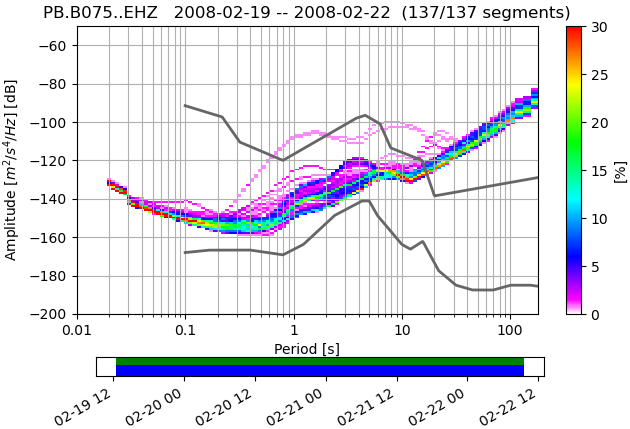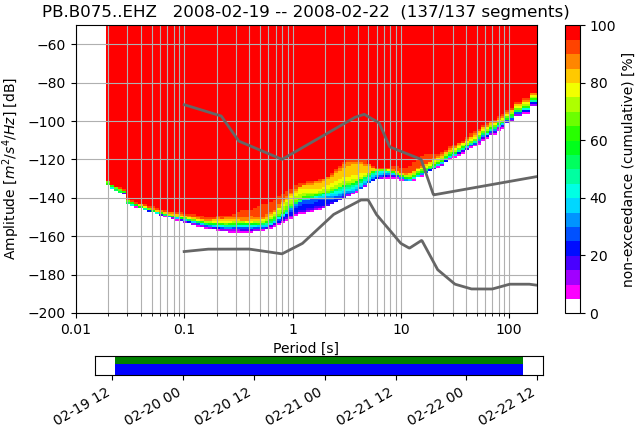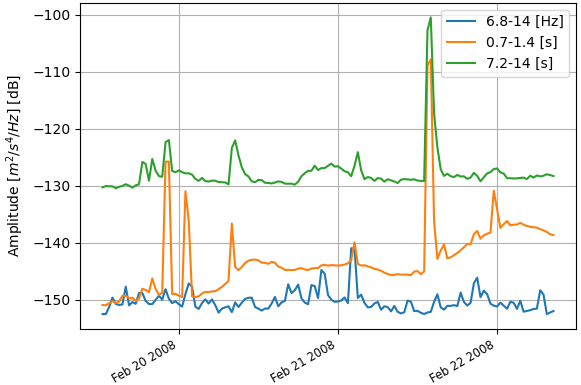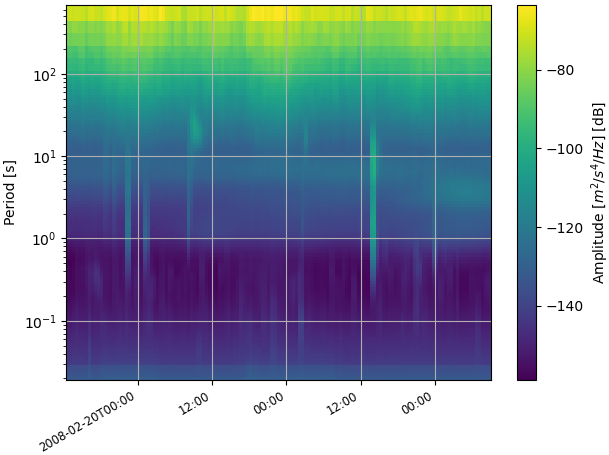1. McNamara, D. E., & Buland, R. P. (2004). Ambient Noise Levels in the Continental United States. Bulletin of the Seismological Society of America, 94(4), 1517–1527.
2. McNamara, D. E., & Boaz, R. I. (2006). Seismic noise analysis system using power spectral density probability density functions: A stand-alone software package. Citeseer.